## ML Aggarwal Class 9 Solutions for ICSE Maths Chapter 12 Pythagoras Theorem Chapter Test

Question 1.
(a) In fig. (i) given below, AD ⊥ BC, AB = 25 cm, AC = 17 cm and AD = 15 cm. Find the length of BC.
(b) In figure (ii) given below, ∠BAC = 90°,
∠ADC = 90°, AD = 6 cm, CD = 8 cm and BC = 26 cm. Find :
(i) AC
(ii) AB
(iii) area of the shaded region.
(c) In figure (iii) given below, triangle ABC is right angled at B. Given that AB = 9 cm, AC = 15 cm and D, E are mid-points of the sides AB and AC respectively, calculate
(i) the length of BC (ii) the area of ∆ADE.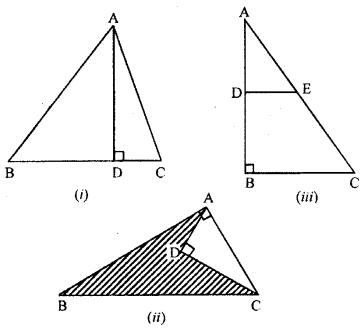Answer: (a) Given. In ∆ ABC , AD ⊥ BC, AB = 25 cm, AC = 17 cm and AD = 15 cm
Required. The length of BC.
Solution:
In right angled ∆ABD,
AB2 = AD2 + BD2 (By Pythagoras theorem)
∴ BD2 = AB2 – AD2
= (25)2 – (15)2
= 625 – 225 = 400
⇒ BD = $$\sqrt{400}$$ = 20 cm.
Now, in right angled ∆ ADC
AC2 = AD2 + DC2 (By Pythagoras theorem)
⇒ DC2 = (17)2 – (15)2
⇒ DC2 = 289 – 225 = 64
DC = $$\sqrt{64}$$ = 8cm
Hence, BC = BD + DC = 20 cm + 8 cm = 28 cm.

(b) Given. In ∆ ABC,
∠BAC = 90°, ∠ ADC = 90° AD = 6cm, CD = 8 cm and BC = 26 cm.
Required. (i) AC (ii) AB
(iii) area of the shaded region
Solution:
AC2 = AD2 + DC2 (By Pythagoras theorem)
= (6)2 + (8)2
= 36 + 64 = 100
∴ = $$\sqrt{10}$$ = 10 cm Answer:
In right angled ∆ ABC
BC2 = AB2 + AC2 (By Pythagoras theorem)
⇒ (26)2 = AB2 + (10)2
⇒ AB2 = (26)2 – (10)2
⇒ AB2 = 676 – 100 = 576
⇒ AB2 = 576
⇒ AB = $$\sqrt{576}$$ = 24 cm
Now, Area of A ABC = $$\frac{1}{2}$$ × AB × AC
= $$\frac{1}{2}$$ × 24 × 10 cm2 = 12 × 10 cm2 = 120 cm2
Area of ∆ ADC = $$\frac{1}{2}$$ × AD × DE
= $$\frac{1}{2}$$ × 6 × 8 cm2 =3 × 8 cm2 = 24 cm2
Now, Area of ∆ ABC = $$\frac{1}{2}$$ × AB × AC
= $$\frac{1}{2}$$ × 24 × 10 cm2 = 12 × 10 cm2 = 120 cm2
Area of ∆ ADC = $$\frac{1}{2}$$ × AD × DC
= $$\frac{1}{2}$$ × 6 × 8 cm2 = 3 × 8 cm2 = 24 cm2
Hence, area of shaded region = Area of ∆ ABC – Area of ∆ ADC = 120 cm2 – 24 cm2 = 96 cm2

(c) Given. In right angled ∆ ABC, AB = 9 cm, AC = 15 cm, and D, E are mid-points of the sides AB and AC respectively.
Required. (i) length of BC (ii) the area of ∆ ADE
Solution:
In right angled ∆ ADE, (By Pythagoras theorem)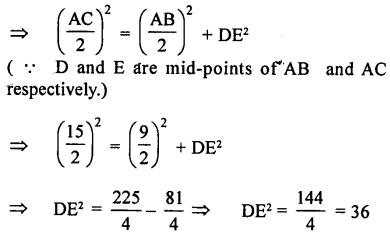Question 2.
If in ∆ ABC, AB > AC and AD ⊥ BC, prove that AB2 – AC2 = BD2 – CD2.
Given. In ∆ ABC, AB > AC and AD ⊥ BC
To prove. AB2 – AC2 = BD2 – CD2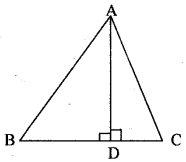Proof. In right angled ∆ ABD
AB2 = AD2 + BD2 …….(1) (By Pythagoras theorem
In right angled ∆ACD
AC2 = AD2 + CD2 ……(2)
Subtracting (2) from (1), we get
= AD2 + BD2 – AD2 – CD2 = BD2 – CD2
∴ AB2 – AC2 = BD2 – CD2 Hence, the result.

Question 3.
In a right angled triangle ABC, right angled at C, P and Q are the points on the sides CA and CB respectively which divide these sides in the ratio 2 : 1. Prove that
(i) 9AQ2 = 9AC2 + 4BC2
(ii) 9BP2 = 9BC2 + 4AC2
(iii) 9(AQ2 + BP2) = 13AB2.
Solution:
Given. A right angled ∆ ABC in which ∠C = 90°. P and Q are points on the side CA and CB respectively such that CP : AP = 2 : 1 and CQ : BQ = 2 : 1
To prove. (i) 9AQ2 = 9AC2 + 4BC2
(ii) 9BP2 = 9BC2 + 4AC2
(iii) 9 (AQ2 + BP2) = 13 AB2Construction. Join AQ and BP.
Proof. (i) In right angled ∆ ACQ
AQ2 = AC2 + QC2 (By Pythagoras theorem)
9AQ2 = 9AC2 + 9QC2 ( Multiplying both sides by 9)
= 9AC2 + (3QC)2 = 9AC2 + (2BC)2= 9AC2 + 4BC2
∴ 9AQ2 = 9AC2 + 4BC2 …..(1)

(ii) In right angled ∆ BPC
BP2 = BC2 + CP2 (By Pythagoras theorem)
9BP2 = 9BC2 + 9CP2 (∵ Multiplying both side by 9)
= 9BC2 + (3 CP)2 = 9BC2 + (2AC)2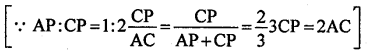= 9BC2 + 4AC2
∴ 9BP2 = 9BC2 + 4AC2 ….(2)

9AQ2 + 9BP2 = 9AC2 + 4BC2 + 9BC2 + 4AC2
= 13AC2 + 13BC2 = 13 (AC2 + BC2) = 13 AB2 [In right angled ∆ ABC = AB2 = AC2 + BC2]
∴ 9AQ + 9BP2 = 13 AB2
Hence, the result.

Question 4.
In the given figure, ∆PQR is right angled at Q and points S and T trisect side QR. Prove that 8PT2 = 3PR2 + 5PS2.Solution:
In the ∆PQR, ∠Q = 90°
T and S are points on RQ such that these trisect it
i.e., RT = TS = SQ
To prove : 8PT2 = 3PR2 + 5PS2
Proof: Let RT = TS = SQ = x
In right ∆PRQ
PR2 = RQ2 + PQ2 = (3x)2 + PQ2 = 9x2 + PQ2
Similarly in right PTS,
PT2 = TQ2 + PQ2 = (2x)2 + PQ2 = 4x2 + PQ2
and in PSQ,
PS2 = SQ2 + PQ2 = x2 + PQ2
8PT2 = 8(4x2 + PQ2) = 32x2 + 8PQ2
3PR2 = 3(9x2 + PQ2) = 27x2 + 3PQ2
5PS2 = 5(x2 + PQ2) = 5x2 + 5PQ2
LHS = 8PT2 = 32x2 + 8PQ2
RHS = 3PR2 + 5PS2 = 27x2 + 3PQ2 + 5x2 + 5PQ2.
= 32x2 + 8PQ2.
LHS = RHS
Hence proved.

Question 5.
In a quadrilateral ABCD, ∠B = 90°. If AD2 = AB2 + BC2 + CD2, prove that ∠ACD = 90°.
Solution:
In quadrilateral ABCD, ∠B = 90° and AD2 = AB2 + BC2 + CD2
To prove : ∠ACD = 90°
Proof: In ∠ABC, ∠B = 90°
∴ AC2 = AB2 + BC2 …(i) (Pythagoras Theorem)
But AD2 = AB2 + BC2 + CD2 (Given)⇒ AD2 = AC2 + CD2 [From (i)]
∴ In ∆ACD,
∠ACD = 90° (Converse of Pythagoras Theorem)

Question 6.
In the given figure, find the length of AD in terms of b and c.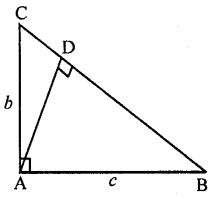Solution:
In the given figure,
ABC is a triangle, ∠A = 90°
AB = c, AC = b
To find : AD in terms of b and c
Solution: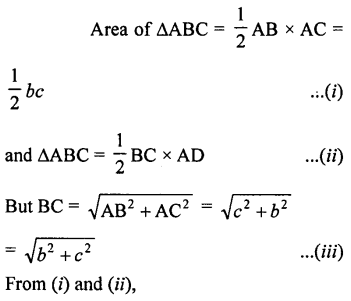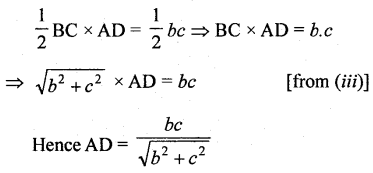Question 7.
ABCD is a square, F is mid-point of AB and BE is one-third of BC. If area of ∆FBE is 108 cm2, find the length of AC.
Solution:
Given : In square ABCD. F is mid piont of
AB and BE = $$\frac{1}{3}$$ BC
Area of AFBE = 108 cm2
AC and EF are joinedTo find: AC
Solution:
Let each side of square is = a
FB = $$\frac{1}{2}$$ AB (F is mid point of AB)
= $$\frac{1}{2}$$ a
and BE = $$\frac{1}{3}$$BC = $$\frac{1}{3}$$a
Now in square ABCD
AC = $$\sqrt{{2}}$$ × Side = $$\sqrt{{2}}$$a
and area ∆FBE = $$\frac{1}{2}$$ FB × BE
= $$\frac{1}{2}$$ × $$\frac{1}{2}$$a × $$\frac{1}{2}$$a = $$\frac{1}{12}$$a2
∴ $$\frac{1}{12}$$ a2 = 108 ⇒ a2 = 12 × 108 = 1296
⇒ a = $$\sqrt{{1296}}$$ = 36
∴ AC = $$\sqrt{{2}}$$ a = $$\sqrt{{2}}$$ × 36 = 36 $$\sqrt{{2}}$$ cm

Question 8.
In a triangle ABC, AB = AC and D is a point on side AC such that BC2 = AC × CD. Prove that BD = BC.
Solution:
Given. In a triangle ABC, AB = AC and D is point on side AC such that BC2 = AC × CD
To prove. BD = BC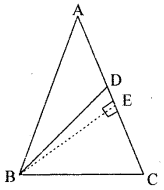Construction. Draw BE ⊥ AC
Proof. In right angled ∆ BCE
BC2 = BE2 + EC2 (By Pythagoras theorem)
= BE2 + (AC – AE)2
= BE2 + AC2 + AE2 -2AC.AE
= (BE2 + AE2) + AC2 – 2AC.AE
= AB2 + AC2 – 2AC.AE (In rt. ∠ ed ∆ ABC, AB2 = BE2 + AE2)
= AC2 + AC2 – 2AC.AE (given AB = AC)
= 2AC2 – 2AC. AE = 2AC (AC – AE)
= 2AC.EC
But BC2 = AC × CD (given)
⇒ AC × CD = 2AC.EC ⇒ CD = 2EC
∴ E is mid-points of CD ⇒ EC = DE
Now, in ∆BED and ∆ BEC
EC = DE (above proved)
BE = BE (common)
∠ BED = ∠ BEC (each 90°)
∴ ∆BED ≅ ∆ BEC (By S. A. S. axiom of congruency)
∴ BD = BC (c.p.c.t.)
Hence, the result.